# C++类的介绍

### 1.类的定义：

C++一个类定义的形式如下：
class 类名
{
成员列表
};

1.每个类可以没有成员，也可以有多个成员。
2.类成员可以是数据或函数。
3.所有成员必须在类内部声明，一旦类定义完成后，就没有任何其他方式可以再增加或减少成员。

class 类名
{
返回类型   函数名(形参列表)
{
函数体
}
};

class 类名
{
返回类型   函数名(形参列表);
};

函数体

### 2.类成员的访问控制：

class 类名
{
public:
公有的数据成员和成员函数
protected:
保护的数据成员和成员函数
private:
私有的数据成员和成员函数
};

class Test
{
public:
void Sum(int a=0,int b=0);
};
void Test::Sum(int a=0,int b=0)
{
cout<<a+b;
}

class Test
{
public:
void Sum(int a=0,int b=0);
};
void Test::Sum(int a,int b)
{
cout<<a+b;
}

### 3.对象的定义和使用：

Test test1 , test2;
class Test test1 , test2;

Test *p;
p = new Test;

delete p;

Test test;
test.Sum();

Test *p;
p = new Test;
p->Sum();

Test test, &r = test;
r.Sum();

### 4.构造函数与析构函数：

class  Test
{
int x = 0;
...
};

C++规定构造函数的名字要与类名保持一致，而且不能指定返回类型。请看下面程序：
#include <iostream>

using namespace std;

class Test
{
public:
Test ();
Test (int x,int y);
void Sum();
private:
int a,b;
};
Test::Test()
{

}
Test::Test(int x,int y)
{
a=x;
b=y;
}
void Test::Sum()
{
cout<<a+b;
}
int main()
{
Test test(3,4);
test.Sum();
return 0;
}


Test test;

Test test;

#include <iostream>

using namespace std;

class Test
{
public:
Test ();
Test (int x,int y);
void Sum();
private:
int a,b;
};
Test::Test()
{

}
Test::Test(int x,int y):a(x),b(y)
{

}
void Test::Sum()
{
cout<<a+b;
}
int main()
{
Test test(3,4);
test.Sum();
return 0;
}

#include <iostream>

using namespace std;

class Test
{
public:
Test () {}
Test (int x,int y):a(x),b(y) {}
void Sum();
private:
int a,b;
};

void Test::Sum()
{
cout<<a+b;
}
int main()
{
Test test(3,4);
test.Sum();
return 0;
}

#include <iostream>

using namespace std;

class Test
{
public:
Test () {}
Test (int x,int y):a(x),b(y) {}
void Sum();
private:
int a,b;
};

void Test::Sum()
{
cout<<a+b;
}
class AnotherTest
{
public:
AnotherTest(int i,int j):test(i,j) {test.Sum();}
private:
Test test;
};
int main()
{
AnotherTest test(3,4);
return 0;
}


#include <iostream>

using namespace std;

class Test
{
public:
Test () {}
Test (int x = 0,int y = 0):a(x),b(y) {}
void Sum();
private:
int a,b;
};

void Test::Sum()
{
cout<<a+b;
}

int main()
{
Test test(3);
test.Sum();
return 0;
}

Test (int x = 0,int y):a(x),b(y) {}

Test test1(3,4);
Test test2 = test1;

{
函数体
}

#include <iostream>

using namespace std;

class Test
{
public:
Test () {}
Test (int x ,int y):a(x),b(y) {}
Test (const Test& t):a(t.a),b(t.b) {}
void Sum();
private:
int a,b;
};

void Test::Sum()
{
cout<<a+b;
}

int main()
{
Test test1(3,4);
Test test2 = test1;
test2.Sum();
return 0;
}


Test test2(test1);

#include <iostream>
#include <cstring>

using namespace std;

class Test
{
public:
Test (int x,char *ptr)
{
a = x;
p = new char [x];
strcpy(p,ptr);
}
Test (const Test& C)
{
a = C.a;
p = new char [a];
p = C.p;
}
void Print();
private:
int a;
char *p;
};

void Test::Print()
{
int i = 0;
while(p[i] != '\0')
{
cout<<p[i];
i++;
}

}

int main()
{
char p = "test";
Test a(10,p);
Test b(a);
b.Print();
return 0;
}


Test (const Test& C)
{
a = C.a;
p = new char [a];
if(p != 0)
strcpy(p,C.p);
}

#include <iostream>
#include <cstring>

using namespace std;

class Test
{
public:
Test (int x,char *ptr)
{
a = x;
p = new char [x];
strcpy(p,ptr);
}
Test (const Test& C)
{
a = C.a;
p = new char [a];
if(p != 0)
strcpy(p,C.p);
}
~Test()
{
delete (p);
cout<<"p has been destroyed"<<endl;
}
void Print();
private:
int a;
char *p;
};

void Test::Print()
{
int i = 0;
while(p[i] != '\0')
{
cout<<p[i];
i++;
}
cout<<endl;
}

int main()
{
char p = "test";
Test a(10,p);
Test b(a);
b.Print();
return 0;
}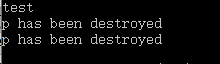### 5.友元机制：

C++提供了友元机制，允许一个类将其非公有成员的访问权限授予指定的函数或类。友元的声明只能在类定义的内部，因此，访问类非公有成员除了自身成员，还有友元。

#include <iostream>
#include <cstring>

using namespace std;

class Test
{
public:
Test (int a)
{
x = a;
}
~Test()  //析构函数
{

}
friend void Print(Test& a,Test& b);
private:
int x;
};

void Print(Test& a,Test& b)
{
cout<<a.x*b.x;
}

int main()
{
Test a(10);
Test b(3);
Print(a,b);
return 0;
}


#include <iostream>
#include <cstring>

using namespace std;

class B;  //类的前向声明
class A
{
public:
A(){}
~A()  //析构函数
{

}
void Print(B& a);
};

class B
{
public:
B (int a)
{
x = a;
}
private:
int x;
friend void A::Print(B& a);
};
void A::Print(B& a)
{
cout<<a.x;
}
int main()
{
B test1(3);
A test2;
test2.Print(test1);
return 0;
}

类A成功的访问了类B的私有成员，并且打印出来。输出结果为 3。

### 6.继承与派生：

#include <iostream>

using namespace std;

class Parallelogram
{
public:
Parallelogram(int a,int b):length(a),width(b) {}
int getLength(){return length;}
int getWidth() {return width;}
private:
int length,width;
};
class Rectangle : public Parallelogram  //公有继承
{
public:
Rectangle(int a,int b):Parallelogram(a,b) {}  //先对基类中的数据成员进行初始化
void Area()     //计算面积
{
cout<<getLength()*getWidth();
}
};
int main()
{
Rectangle r(3,4);
r.Area();
return 0;
}


public(公有继承)，基类中的公有和保护成员保持原属性，私有成员为基类私有。
private(私有继承)，基类中的所有成员在派生类中都是私有的。
protected(保护继承)，基类的公有成员和保护成员在派生类中成了保护成员，私有成员仍为基类私有。

①从基类接收成员，除了构造函数和析构函数，派生类会把全部的成员继承过来，这是没有选择的。
②调整基类成员的访问。
③修改基类成员，可以在派生类中声明一个与基类同名的成员，此操作会覆盖基类的同名成员。
④在定义派生类的时候定义新的成员，定义构造函数和析构函数，初始化的时候必须先将基类的成员初始化(因为并没有继承基类的构造函数)之后才可以对派生类的成员进行初始化。析构函数也一样，需要在派生类中释放基类的数据成员(调用基类的析构函数)。

C++还支持一个派生类同时继承多个基类。

class  派生类名 : 访问标号1 基类名1 , 访问标号2 基类名2 , ....

{

成员列表

}

#include <iostream>

using namespace std;

class BaseOne
{
public:
BaseOne() {cout<<"This is BaseOne"<<endl;}
BaseOne(int a):data(a) {cout<<"BaseOne's data is "<<data<<endl;}
private:
int data;
};

class BaseTwo
{
public:
BaseTwo() {cout<<"This is BaseTwo"<<endl;}
BaseTwo(int a):data(a) {cout<<"BaseTwo's data is "<<data<<endl;}
private:
int data;
};

class BaseThree
{
public:
BaseThree() {cout<<"This is BaseThree"<<endl;}
};

class Derive:public BaseOne,public BaseTwo,public BaseThree
{
public:
Derive () {cout<<"This is Derive"<<endl;}
Derive (int a,int b,int c,int d,int e):BaseOne(a),BaseTwo(b),dataOne(c),dataTwo(d),data(e)
{cout<<"Derive's data is "<<data<<endl;}
private:
BaseOne dataOne;
BaseTwo dataTwo;
int data;
};

int main()
{
Derive r1;
cout<<endl;
Derive r2(1,2,3,4,5);
return 0;
}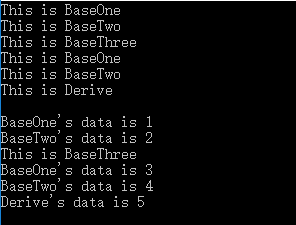#include <iostream>
#include <cstring>

using namespace std;

class A
{
public:
void fun() {cout<<"This is A"<<endl;}
};

class B
{
public:
void fun() {cout<<"This is B"<<endl;}
};

class C:public A,public B
{
public:
void hun() {fun();}  //产生二义性
};

int main()
{
C c;
c.hun();
return 0;
}


void hun() {A::fun(); B::fun();}

#include <iostream>

using namespace std;

class A
{
public:
void fun() {cout<<"This is A"<<endl;}
};

class B:public A
{
public:
void gun() {cout<<"This is B"<<endl;}
};

class C:public A
{
public:
void hun() {cout<<"This is C"<<endl;}
};

class D:public B,public C
{
public:
void kun() {fun();} //产生二义性
};

int main()
{
D d;
d.kun();
return 0;
}


A 是基类，B 是 A 的派生类，C 也是 A 的派生类，而 D 是 B 和 C 的派生类，因此 D 可以访问 A 的数据成员，但现在会产生二义性问题，我们必须显式的指出 fun() 是来自 B 的 还是来自 C 的，但是我们都知道它来自 A , 因此我们希望找到一种方式，使得在继承间接共同基类时只保留一份成员，这就用到了虚基类的机制。

class  派生类名 : virtual  访问标号 虚基类名 , ...

{

成员列表

class B:virtual public A

class C:virtual public A

#include <iostream>

using namespace std;

class A
{
public:
A() {cout<<"This is Grandpa"<<endl;}
A(int a):One(a) {cout<<"Grandpa is "<<One<<" years old"<<endl;}
~A() {cout<<"A is over"<<endl;}
private:
int One;
};

class B:virtual public A
{
public:
B() {cout<<"This is father"<<endl;}
B(int a,int b):A(a),Two(b) {cout<<"father is "<<Two<<" years old"<<endl;}
~B() {cout<<"B is over"<<endl;}
private:
int Two;
};

class C:virtual public A
{
public:
C() {cout<<"This is mother"<<endl;}
C(int a,int b):A(a),Three(b) {cout<<"mother is "<<Three<<" years old"<<endl;}
~C() {cout<<"C is over"<<endl;}
private:
int Three;
};

class D:public B,public C
{
public:
D() {cout<<"This is me"<<endl;}
D(int a,int b,int c,int d):A(a),B(a,b),C(a,c),Four(d) {cout<<"I am "<<Four<<" years old"<<endl;}
~D() {cout<<"D is over"<<endl;}
private:
int Four;
};

int main()
{
D d1;
cout<<endl;
//D d2(65,40,39,13);
return 0;
}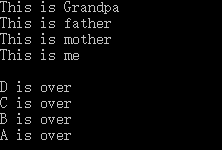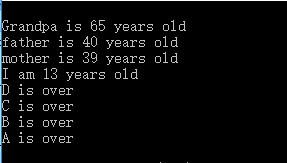### 7.多态性和虚函数：

#include <iostream>

using namespace std;

class A
{
public:
void fun() {cout<<"Use A"<<endl;}
};

class B : public A
{
public:
void fun() {cout<<"Use B"<<endl;}
};

int main()
{
B b;
A *p = &b;
p->fun();
return 0;
}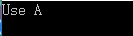virtual void fun() {cout<<"Use A"<<endl;}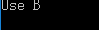virtual   返回类型  函数名 (形式参数列表) = 0;

#include <iostream>

using namespace std;

class Sharp
{
public:
virtual double area() = 0;
virtual double volumn() = 0;
};

class Circle : public Sharp
{
public:
Circle(double r):R(r) {}
virtual double area() {return 3.1415926*R*R;}
virtual double volumn() {return 0;}
private:
double R;
};

class Cylinder : public Circle
{
public:
Cylinder(double a,double b):Circle(a),H(b) {}
virtual double volumn() {return area()*H;}
private:
double H;
};

int main()
{
Circle a(20.0);
Cylinder b(10.0,2.0);
cout<<a.area()<<endl;
cout<<b.volumn()<<endl;
return 0;
}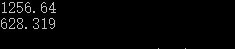06-071653

#### C ++类与对象08-232万+

#### C++中的类——类的定义和声明

08-015万+

#### C++类(Class)的定义与实现

02-092万+

#### C++ 类详解

04-192万+

#### C++中的 ::

07-039761

#### C++：C++11的牛逼特性

05-047323

#### C++类大小详尽讲解

11-111106

#### 对C++的一些介绍(类的性质)©️2020 CSDN 皮肤主题: 技术黑板 设计师: CSDN官方博客点击重新获取扫码支付1.余额是钱包充值的虚拟货币，按照1:1的比例进行支付金额的抵扣。
2.余额无法直接购买下载，可以购买VIP、C币套餐、付费专栏及课程。余额充值